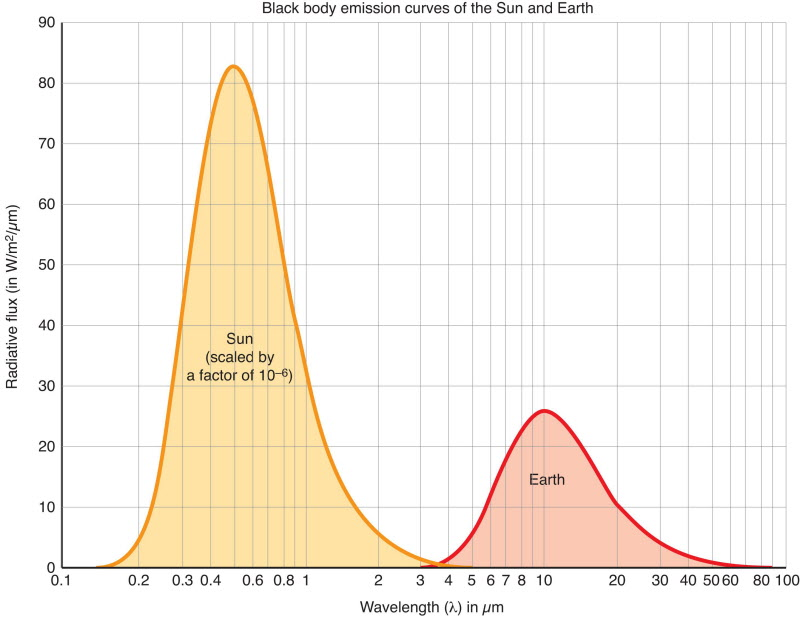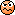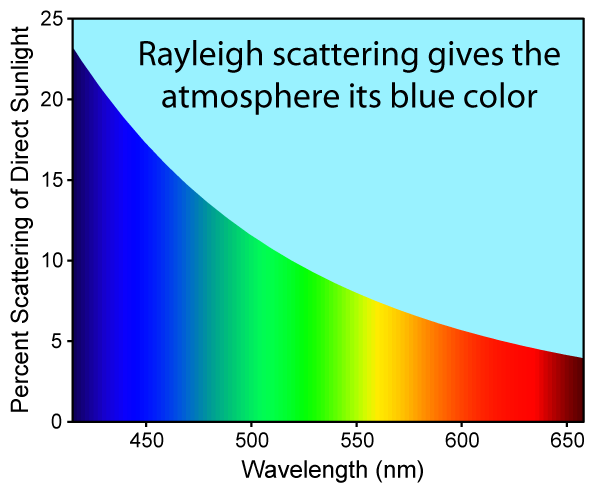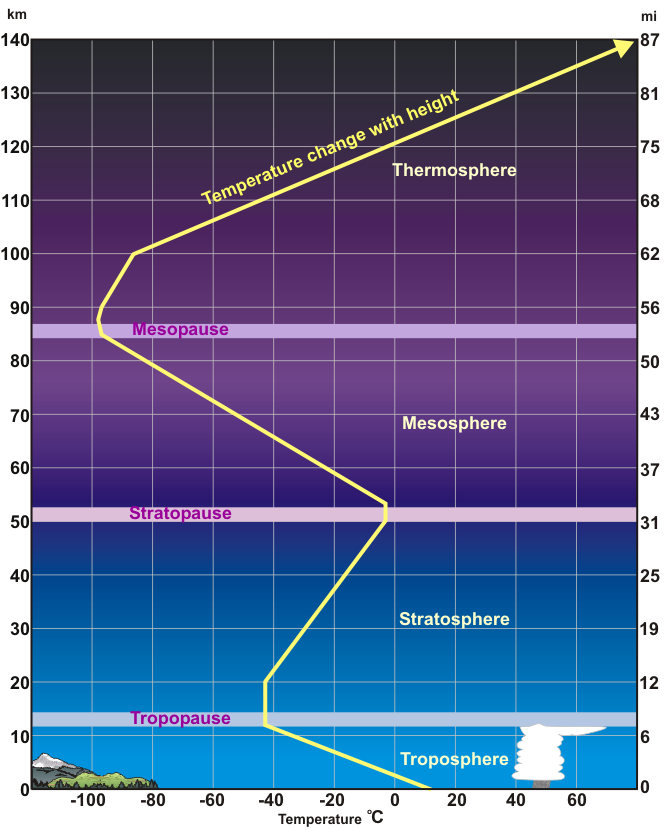# The Azimuth Project Blog - mathematics of the environment (part 3) (changes)

Showing changes from revision #0 to #1: Added | Removed | Changed

This page is a blog article in progress, written by John Baez To discuss it, go to the Azimuth Forum. For the final polished version, visit the Azimuth Blog.

This week I’ll release these notes before my seminar, so my students (and all of you, too) can read them ahead of time. The reason is that I’m pushing into topics I don’t understand as well as I’d like. So, my notes wrestle with some ideas in too much detail to cover in class—and I’m hoping some students will look at these notes ahead of time to prepare. Also, I’d appreciate your comments!

This week I’ll borrow material shamelessly from here:

• Seymour L. Hess, Introduction to Theoretical Meteorology, Henry Holt and Company, New York, 1959.

It’s an old book: for example, it talks about working out the area under a curve using a gadget called a planimeter, which is what people did before computers.It also talks about how people measured the solar constant (roughly, the brightness of the Sun) before we could easily put satellites up above the Earth’s atmosphere! And it doesn’t mention global warming.

But despite or perhaps even because of these quaint features, it’s simple and clear. In case it’s not obvious yet, I’m teaching this quarter’s seminar in order to learn stuff. So, I’ll sometimes talk about old work… but if you catch me saying things that are seriously wrong (as opposed to merely primitive), please let me know.

### The plan

Last time we considered a simple model Earth, a blackbody at uniform constant temperature absorbing sunlight and re-emitting the same amount of power in the form of blackbody radiation. We worked out that its temperature would be 6 °C, which is not bad. But then we took into account the fact that the Earth is not black. We got a temperature of -18 °C, which is too cold. The reason is that we haven’t yet equipped our model Earth with an atmosphere! So today let’s try that.

At this point things get a lot more complicated, even if we try a 1-dimensional model where the temperature, pressure and other features of the atmosphere only depend on altitude. So, I’ll only do what I can easily do. I’ll explain some basic laws governing radiation, and then sketch how people applied them to the Earth.

It’ll be good to start with a comment about what we did last time.

### Kirchoff's law of radiation

When we admitted the Earth wasn’t black, we said that it absorbed only about 70% of the radiation hitting it… but we still modeled it as emitting radiation just like a blackbody! Isn’t there something fishy about this?

Well, no. The Earth is mainly absorbing sunlight at visible frequencies, and at these frequencies it only absorbs about 70% of the radiation that hits it. But it mainly emits infrared light, and at these frequencies it acts like it’s almost black. These frequencies are almost completely different from those where absorption occurs.But still, this issue is worth thinking about.

After all, emission and absorption are flip sides of the same coin. There’s a deep principle in physics, called reciprocity, which says that how X affects Y is not a separate question from how Y affects X. In fact, if you know the answer to one of these questions, you can figure out the answer to the other!The first place most people see this principle is Newton’s third law of classical mechanics, saying that if X exerts a force on Y, Y exerts an equal and opposite force on X.

For example: if I punched your nose, your nose punched my fist just as hard, so you have no right to complain.This law is still often stated in its old-fashioned form:

For every action there is an equal and opposite reaction.

I found this confusing as a student, because ‘force’ was part of the formal terminology of classical mechanics, but not ‘action’—at least not as used in this sentence!—and certainly not ‘reaction’. But as a statement of the basic intuition behind reciprocity, it’s got a certain charm.

In engineering, the principle of reciprocity is sometimes stated like this:

Reciprocity in linear systems is the principle that the response $R_{ab}$ measured at a location $a$ when the system is excited at a location $b$ is exactly equal to $R_{ba},$ which is the response at location $b$ when that same excitation is applied at $a.$ This applies for all frequencies of the excitation.

Again this is a bit confusing, at least if you’re a mathematician who would like to know exactly how a ‘response’ or an ‘excitation’ is defined. It’s also disappointing to see the principle stated in a way that limits it to linear systems. Nonetheless it’s tremendously inspiring. What’s really going on here?

I don’t claim to have gotten to the bottom of it. My hunch is that to a large extent it will come down to the fact that mixed partial derivatives commute. If we’ve got a smooth function $f$ of a bunch of variables $x_1, \dots, x_n,$ and we set

$\displaystyle{ R_{ab} = \frac{\partial^2 f}{\partial x_a \partial x_b} }$

then

$R_{ab} = R_{ba}$

However, I haven’t gotten around to showing that reciprocity boils down to this in all the examples yet. Yet another unification project to add to my list!

Anyway: reciprocity has lots of interesting applications to electromagnetism. And that’s what we’re really talking about now. After all, light is electromagnetic radiation!

The simplest application is one we learn as children:

If I can see you, then you can see me.

or at least:

If light can go from X to Y in a static environment, it can also go from Y to X.

But we want something that sounds a bit different. Namely:

The tendency of a substance to absorb light at some frequency equals its tendency to emit light at that frequency.

This is too vague. We should make it precise, and in a minute I’ll try, but first let me motivate this idea with a thought experiment. Suppose we have a black rock and a white rock in a sealed mirrored container. Suppose they’re in thermal equilibrium at a very high temperature, so they’re glowing red-hot. So, there’s red light bouncing around the container. The black rock will absorb more of this light. But since they’re in thermal equilibrium, the black rock must also be emitting more of this light, or it would gain energy and get hotter than the white one. That would violate the zeroth law of thermodynamics, which implies that in thermal equilibrium, all the parts of a system must be at the same temperature.

More precisely, we have:

Kirchoff’s Law of Thermal Radiation. For any body in thermal equilibrium, its emissivity equals its absorptivity.

Let me explain. Suppose we have a surface made of some homogeneous isotropic material in thermal equilibrium at temperature $T.$ If it’s perfectly black, we saw last time that it emits light with a monochromatic energy flux given by the Planck distribution:

$\displaystyle{ f_{\lambda}(T) = \frac{2 hc^2}{\lambda^5} \frac{1}{ e^{\frac{hc}{\lambda k T}} - 1 } }$

Here $\lambda$ is the wavelength of light and the ‘monochromatic energy flux’ has units of power per area per wavelength.

But if our surface is not perfectly black, we have to multiply this by a fudge factor between 0 and 1 to get the right answer. This factor is called the emissivity of the substance. It can depend on the wavelength of the light quite a lot, and also on the surface’s temperature (since for example ice melts at high temperatures and gets darker). So, let’s call it $e_\lambda(T).$

We can also talk about the absorptivity of our surface, which is the fraction of light it absorbs. Again this depends on the wavelength of the light and the temperature of our surface. So, let’s call it $a_\lambda(T).$

Then Kirchoff’s law of thermal radiation says

$e_\lambda(T) = a_\lambda(T)$

So, for each frequency the emissivity must equal the absorptivity… but it’s still possible for the Earth to have an average emissivity near 1 at the wavelengths of infrared light and near 0.7 at the wavelengths of visible light. So there’s no paradox.

Puzzle 1. Is this law named after the same guy who discovered Kirchhoff’s laws governing electrical circuits?

### Schwarzschild's equation

Now let’s talk about light shining through the Earth’s atmosphere. Or more generally, light shining through a medium. What happens? It can get absorbed. It can get scattered, bouncing off in different directions. Light can also get emitted, especially if the medium is hot. The air in our atmosphere isn’t hot enough to emit a lot of visible light, but it definitely emits infrared light and microwaves.

It sounds complicated, and it is, but there are things we can say about it. Let me tell you about Schwarzschild’s equation.

Light comes in different wavelengths. So, can ask how much power per square meter this light carries per wavelength. We call this the monochromatic energy flux $I_{\lambda},$ since it depends on the wavelength $\lambda.$ As mentioned last time, this has units W/m2μm, where μm stands for micrometers, a unit of wavelength.

However, because light gets absorbed, scattered and emitted the monochromatic energy flux is really a function $I_{\lambda}(s),$ where $s$ is the distance through the medium. Here I’m imagining an essentially one-dimensional situation, like a beam of sunlight coming down through the air when the Sun is directly overhead. We can generalize this later.

Let’s figure out the basic equation describing how $I_{\lambda}(s)$ changes as a function of $s.$ This is called the equation of radiative transfer, or Schwarzschild’s equation. It won’t tell us how different gases absorb different amounts of light of different frequencies—for that we need to do hard calculations, or experiments. But we can feed the results of these calculations into Schwarzschild’s equation.

For starters, let’s assume that light only gets absorbed but not emitted or scattered. Later we’ll include emission, which is very important for what we’re doing: the Earth’s atmosphere is warm enough to emit significant amounts of infrared light (though not hot enough to emit much visible light). Scattering is also very important, but it can be treated as a combination of absorption and emission.

For absorption only, we have the Beer–Lambert law:

$\displaystyle{ \frac{d I_\lambda(s)}{d s} = - a_\lambda(s) I_\lambda(s) }$

In other words, the amount of radiation that gets absorbed per distance is proportional to the amount of radiation. However, the constant of proportionality $a_\lambda (s)$ can depend on the frequency and the details of our medium at the position $s.$ I don’t know the standard name for this constant $a_\lambda (s),$ so let’s call it the absorption rate.

Puzzle 2. Assuming the Beer–Lambert law, show that the intensity of light at two positions $s_1$ and $s_2$ is related by

$\displaystyle{ I_\lambda(s_2) = e^{-\tau} \; I_\lambda(s_1) }$

where the optical depth $\tau$ of the intervening medium is defined by

$\displaystyle{ \tau = \int_{s_1}^{s_2} a_\lambda(s) \, ds }$

So, a layer of stuff has optical depth equal to 1 if light shining through it has its intensity reduced by a factor of $1/e.$

We can go a bit further if our medium is a rather thin gas like the air in our atmosphere. Then the absorption rate is given by

$a_\lambda(s) = k_\lambda(s) \, \rho(s)$

where $\rho(s)$ is the density of the air at the position $s$ and $k_\lambda(s)$ is its absorption coefficient.

In other words, air absorbs light at a rate proportional to its density, but also depending on what it’s made of, which may vary with position. For example, both the density and the humidity of the atmosphere can depend on its altitude.

What about emission? Air doesn’t just absorb infrared light, it also emits significant amounts of it! As mentioned, a blackbody at temperature $T$ emits light with a monochromatic energy flux given by the Planck distribution:

$\displaystyle{ f_{\lambda}(T) = \frac{2 hc^2}{\lambda^5} \frac{1}{ e^{\frac{hc}{\lambda k T}} - 1 } }$

But a gas like air is far from a blackbody, so we have to multiply this by a fudge factor. Luckily, thanks to Kirchoff’s law of radiation, this factor isn’t so fudgy: it’s just the absorption rate $a_\lambda(s).$

Here are we generalizing Kirchoff’s law from a surface to a column of air, but that’s okay because we can treat a column as a stack of surfaces; letting these become very thin we arrive at a differential formulation of the law that applies to absorption and emission rates instead of absorptivity and emissivity. (If you’re very sharp, you’ll remember that Kirchoff’s law applies to thermal equilibrium, and wonder about that. Air in the atmosphere isn’t in perfect thermal equilibrium, but it’s close enough for what we’re doing here.)

So, when we take absorption and also emission into account, Beer’s law gets another term:

$\displaystyle{ \frac{d I_\lambda(s)}{d s} = - a_\lambda(s) I_\lambda(s) + a_\lambda(s) f_\lambda(T(s)) }$

where $T$ is the temperature of our gas at the position $s.$ In other words:

$\displaystyle{ \frac{d I_\lambda(s)}{d s} = a_\lambda(s) ( f_\lambda(T) - I_\lambda(s))}$

This is Schwarzschild’s equation.

Puzzle 3. Is this equation named after the same guy who discovered the Schwarzschild metric in general relativity, describing a spherically symmetric black hole?

### Application to the atmosphere

In principle, we can use Schwarzschild’s equation to help work out how much sunlight of any frequency actually makes it through the atmosphere down to the Earth, and also how much infrared radiation makes it through the atmosphere out to space. But this is not a calculation I can do here today, because it’s very complicated.

If we actually measure what fraction of radiation of different frequencies makes it through the atmosphere, you’ll see why:

Everything here is a function of the wavelength, measured in micrometers. The smooth red curve is the Planck distribution for light coming from the Sun at a temperature of 5325 K. Most of it is visible light, with a wavelength between 0.4 and 0.7 micrometers. The jagged red region shows how much of this gets through—on a clear day, I assume—and you can see that most of it gets through. The smooth bluish curves are the Planck distributions for light coming from the Earth at various temperatures between 210 K and 310 K. Most of it is infrared light, and not much of it gets through.

This, in a nutshell, is what keeps the Earth warmer than the chilly -18 °C we got last time for an Earth with no atmosphere!

This is the greenhouse effect. As you can see, the absorption of infrared light is mainly due to water vapor, and then carbon dioxide, and then other lesser greenhouse gases, mainly methane, nitrous oxide. Oxygen and ozone also play a minor role, but ozone is more important in blocking ultraviolet light. Rayleigh scattering—the scattering of light by small particles, including molecules and atoms—is also important at short wavelengths, because its strength is proportional to $1/\lambda^4.$ This is why the sky is blue!Here the wavelengths are measured in nanometers; there are 1000 nanometers in a micrometer. Rayleigh scattering continues to become more important in the ultraviolet.

But right now I want to talk about the infrared. As you can see, the all-important absorption of infrared radiation by water vapor and carbon dioxide is quite complicated. You need quantum mechanics to predict how this works from first principles. Tim van Beek gave a gentle introduction to some of the key ideas here:

• Tim van Beek, A quantum of warmth, Azimuth, 2 July 2011.

Someday it would be fun to get into the details. Not today, though!

You can see what’s going on a bit more clearly here:

The key fact is that infrared is almost completely absorbed for wavelengths between 5.5 and 7 micrometers, or over 14 micrometers. (A ‘micron’ is just an old name for a micrometer.)

### The work of Simpson

The first person to give a reasonably successful explanation of how the power of radiation emitted by the Earth balances the power of the sunlight it absorbs was George Simpson. He did it in 1928:

• George C. Simpson, Further studies in terrestrial radiation, Mem. Roy. Meteor. Soc. 3 (1928), 1–26.

One year earlier, he had tried and failed to understand this problem using a ‘gray atmosphere’ model where the fraction of light that gets through was independent of its wavelength. If you’ve been paying attention, I think you can see why that didn’t work.

In 1928, since he didn’t have a computer, he made a simple model that treated emission of infrared radiation as follows.

He treated the atmosphere as made of layers of varying thickness, each layer containing 0.03 grams per centimeter2 of water vapor. The Earth’s surface radiates infrared almost as a black body. Part of the power is absorbed by the first layer above the surface, while some makes it through. The first layer then re-radiates at the same wavelengths at a rate determined by its temperature. Half this goes downward, while half goes up. Of the part going upward, some is absorbed by the next layer… and so on, up to the top layer. He took this top layer to end at the stratosphere, since the atmosphere is much drier in the stratosphere.

He did this all in a way that depends on the wavelength, but using a simplified model of how each of these layers absorbs infrared light. He assumed it was:

• completely opaque from 5.5 to 7 micrometers (due to water vapor),

• partly transparent from 7 to 8.5 micrometers (interpolating between opaque and transparent),

• completely transparent from 8.5 to 11 micrometers,

• partly transparent from 11 to 14 micrometers (interpolating between transparent and opaque),

• completely opaque above 14 micrometers (due to carbon dioxide and water vapor).

He got this result, at the latitude 50° on a clear day:

The upper smooth curve is the Planck distribution for a temperature of 280 K, corresponding to the ground. The lower smooth curve is the Planck distribution at 218 K, corresponding to the stratosphere. The shaded region is his calculation of the monochromatic flux emitted into space by the Earth. As you can see, it matches the Planck distribution for the stratosphere where the lower atmosphere is completely opaque in his model—between 5.5 and 7 micrometers, and over 14 micrometers. It matches the Planck distribution for the stratosphere where the lower atmosphere is completely transparent. Elsewhere, it interpolates between the two.

The area of this shaded region—calculated with a planimeter, perhaps?—is the total flux emitted into space.

This is just part of the story: he also took clouds into account, and he did different calculations at different latitudes. He got a reasonably good balance between the incoming and outgoing power. In short, he showed that an Earth with its observed temperatures is roughly compatible with his model of how the Earth absorbs and emits radiation. Note that this is just another way to tackle the problem of predicting the temperature given a model.

Also note that Simpson didn’t quite use the Schwarzschild equation. But I guess that in some sense he discretized it—right?

### And so on

This was just the beginning of a series of more and more sophisticated models. I’m too tired to go on right now.

You’ll note one big thing we’ve omitted: any sort of calculation of how the pressure, temperature and humidity of the air varies with altitude! To the extent we talked about those at all, we treated them as inputs. But for a full-fledged one-dimensional model of the Earth’s atmosphere, we’d want to derive them from some principles. There are, after all, some important puzzles:

Puzzle 4. If hot air rises, why does the atmosphere generally get colder as you go upward, at least until you reach the stratosphere?Puzzle 5. Why is there a tropopause? In other words, why is there a fairly sudden transition 10 kilometers up between the troposphere, where the air is moist, cooler the higher you go, and turbulent, and the stratosphere, where the air is drier, warmer the higher you go, and not turbulent?

There’s a limit to how much we can understand these puzzles using a 1-dimensional model, but we should at least try to make a model of a thin column of air with pressure, temperature and humidity varying as a function of altitude, with sunlight streaming downward and infrared radiation generally going up. If we can’t do that, we’ll never understand more complicated things, like the actual atmosphere.

category: blog